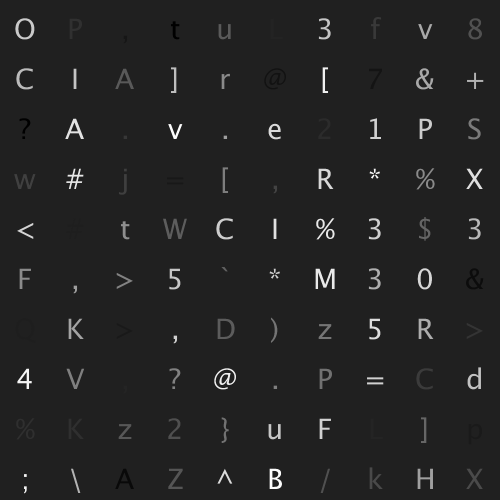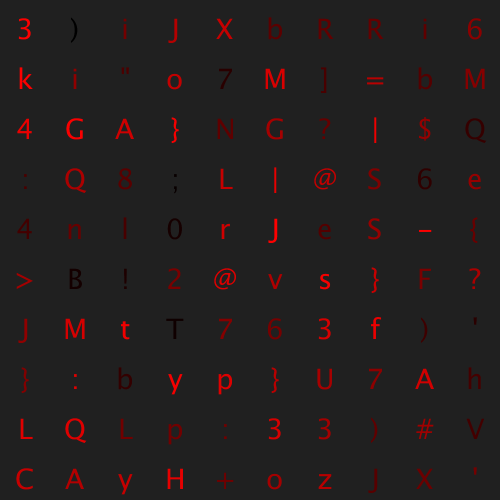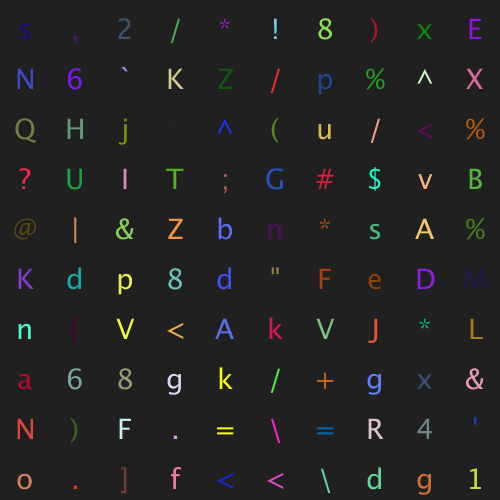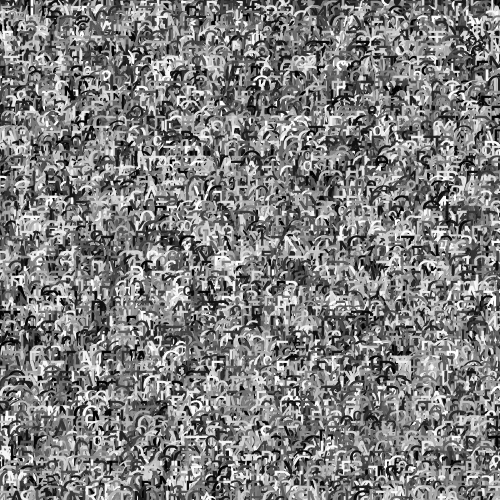# Letters

example processing for-loop typography 100-days-of-code
``````size(500, 500);

int rows = 10;
int cols = 10;

int cellHeight = height/rows;
int cellWidth = width/cols;

textAlign(CENTER, CENTER);
textSize(28);

background(32);

for(int y = 0; y < rows; y++){
for(int x = 0; x < cols; x++){

//get a random ascii letter
char c = '!';
c += random(93);

//calculate cell position
int pixelX = cellWidth * x;
int pixelY = cellHeight * y;

pixelX += cellWidth/2;
pixelY += cellHeight/2;

fill(random(256));
text(c, pixelX, pixelY);
}
}
``````

This code uses nested `for` loops to draw a grid of random letters.Code Editor

See the Pen by Happy Coding (@KevinWorkman) on CodePen.

The code uses the `char` type, which stores an individual letter as an ASCII code. In other words, it stores a letter as a number. This lets us get a random letter by doing this:

``````char c = '!';
c += random(93);
``````

To understand these lines, take a look at the printable characters chart, and notice that `!` is the first printable ascii value, starting with ascii code `33`. There are 93 other printable characters. So adding a random number from 0-93 to the `!` character gives you a random ascii letter!

You could also use the ascii code directly instead of the letter it represents:

``````char c = 33;
c += random(93);
``````

Anyway, this results in a grid of random letters.## Tweak Ideas

• Change the color of the letters. Make them shades of blue, or randomly colored.
• Base the colors of the letters off something other than random. Make A darker than B darker than C, or make letters at the top red and letters at the bottom blue.
• Change the text size as well as the color. Make letters randomly sized, or make vowels bigger, or make symbols a different size.
• Make upper-case letters one color, lower-case letters a different color, and symbols a third color.